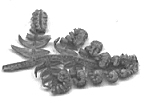# Word Code

## Formatting Numeric Data In A Table

A recent requirement was that someone required to have some numeric data within a table formatted so that the data would have two decimal places and comma thousand dividers.

This meant that a table looking like this:

 1234567.8 1.23457e+06 UB40 1.23457e+06 R2D2 1.23457e+06 1234567.8 1.23457e+06

Would need to be formatted into this:

 1,234,567.80 1,234,567.80 UB40 1,234,567.80 R2D2 1,234,567.80 1,234,567.80 1,234,567.80

Of course anything which was not defined to be numeric should not be formatted. There were a few other implied requirements such as if the selection wasn't in a table then nothing should happen and, of course, there had to be a document open for this code to work.

Below is the code:

```
Option Explicit

Sub FormatTable()

Dim oTable As Table
Dim nRow As Long
Dim nCol As Long
Dim sText As String
Dim bIsNumberic As Boolean

On Error GoTo Error_FormatTable

If Documents.Count > 0 then

Set oTable = Selection.Tables(1)

If Not oTable Is Nothing Then
For nRow = 1 To oTable.Rows.Count
For nCol = 1 To oTable.Columns.Count
sText = oTable.Cell(nRow, nCol).Range.Text
sText = Left\$(sText, Len(sText) - 2)

If IsNumeric(sText) Then
oTable.Cell(nRow, nCol).Range.Text = Format\$(sText, "#,##0.00")
End If

Next nCol
Next nRow
End If

End If

Exit_FormatTable:

Exit Sub

Error_FormatTable:

Resume Exit_FormatTable

End Sub

```

There was the assumption for this project that the numeric data would be formatted in a certain way. We could have added a dialog box, for example, so that the formatting could be defined but this is beyond the scope of this example. But, if this project were to be transformed into a proper shrink-wrapped piece of code then these considerations would have to be undertaken.

The code takes place all within one sub-routine. The first thing to note is that the error handling is going to be required here so we have started off with an On Error statement which will handle all errors. There is one obvious and possible error; that is that the user has selected a part of the document which is not within a table.

If this error, or any other, occurs then control would be moved to the error handling section of the code and then with the Resume statement the error is cleared and then the routine is left.

Right after the instruction for the error handler the number of Documents are counted. If there is at least one document open then we can process this routine further. To check that there is an open document then we simply .Count the number of items within the Documents collection.

In this project we are only concerned with the first table within the selection. We could have changed the code easily so that each table within the selection was processed but that wasn't the case here. Once we have got the pointer to this first table, oTable, then we count the number of rows and columns in the table (by again using .Count in each of the .Rows and .Columns collection. Once we have done this simple set of nested loops allows us to access each cell of the table in turn.

The next thing to do would be to extract the text from the cell. So what we are looking for is the .Text of the .Range of the .Cell. In Word there are two bytes at the end of each text item in a cell. If there is an empty cell then there still would be these two terminating bytes. So, the next stage is obvious; we have to strip the right hand pair of characters from the string. This is done by the Left\$() function.

All that is left is to determine whether this string of text can be identified as a numeric string. This is done by the IsNumeric() function and if the data is determined to be numeric then the range of the text in the cell is formatted as per the requirements.

This code can be downloaded from here and placed into Word's start-up folder.